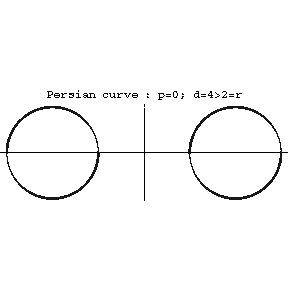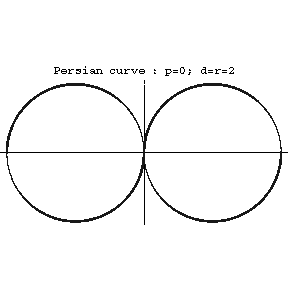# Persian curve

spiric curve

A plane algebraic curve of order four that is the line of intersection between the surface of a torus and a plane parallel to its axis (see Fig. a, Fig. b, Fig. c). The equation in rectangular coordinates is

$$(x^2+y^2+p^2+d^2-r^2)^2=4d^2(x^2+p^2),$$

where $r$ is the radius of the circle describing the torus, $d$ is the distance from the origin to its centre and $p$ is the distance from the axis of the torus to the plane. The following are Persian curves: the Booth lemniscate, the Cassini oval and the Bernoulli lemniscate.Figure: p072400a

$d>r$.Figure: p072400b

$d=r$.Figure: p072400c

$d<r$.

The name is after the Ancient Greek geometer Persei (2nd century B.C.), who examined it in relation to research on various ways of specifying curves.